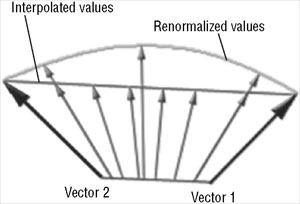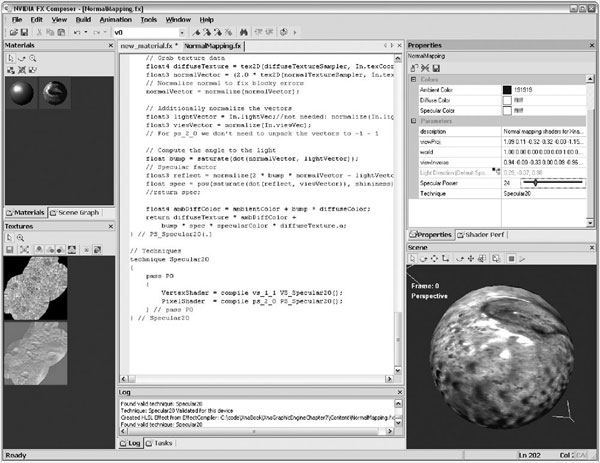• 矩阵（world，worldviewproj，viewinverse）
• 其他的全局变量如时间、测试值等
• 材质数据，首先是材质的颜色，然后是所有使用的材质与采样器
• 顶点结构，最重要的是vertexinput结构，通常使用你已经定义在引擎中的tangentvertex，通常使用如 //-----------------的注释行为每个technique分隔数据块。

// Vertex input structure (used for ALL techniques here!)

struct VertexInput {

float3 pos : POSITION;

float2 texCoord : TEXCOORD0;

float3 normal : NORMAL;

float3 tangent : TANGENT;

};

// Common functions

float4 TransformPosition(float3 pos)//float4 pos)

{

return mul(mul(float4(pos.xyz, 1), world), viewProj);

} // TransformPosition(.)

float3 GetWorldPos(float3 pos)

{

return mul(float4(pos, 1), world).xyz;

} // GetWorldPos(.)

float3 GetCameraPos()

{

return viewInverse.xyz;

}

// GetCameraPos() float3 CalcNormalVector(float3 nor)

{

return normalize(mul(nor, (float3x3)world));

} // CalcNormalVector(.)

// Get light direction

float3 GetLightDir()

{

return lightDir;

} // GetLightDir()

float3x3 ComputeTangentMatrix(float3 tangent, float3 normal)

{

// Compute the 3x3 tranform from tangent space to object space

float3x3 worldToTangentSpace;

worldToTangentSpace = mul(cross(normal, tangent), world);

worldToTangentSpace = mul(tangent, world);

worldToTangentSpace = mul(normal, world);

return worldToTangentSpace;

} // ComputeTangentMatrix(..)

struct VertexOutput_Specular20

{

float4 pos : POSITION;

float2 texCoord : TEXCOORD0;

float3 lightVec : TEXCOORD1;

float3 viewVec : TEXCOORD2;

};

VertexOutput_Specular20 VS_Specular20(VertexInput In)

{

VertexOutput_Specular20 Out = (VertexOutput_Specular20) 0;

Out.pos = TransformPosition(In.pos);

// We can duplicate texture coordinates for diffuse and Normal Map

// in the Pixel Shader 2.0.

Out.texCoord = In.texCoord;

// Compute the 3x3 tranform from tangent space to object space

float3x3 worldToTangentSpace = ComputeTangentMatrix(In.tangent, In.normal);

float3 worldEyePos = GetCameraPos();

float3 worldVertPos = GetWorldPos(In.pos);

// Transform light vector and pass it as a color (clamped from 0 to 1)

// For ps_2_0 we don't need to clamp from 0 to 1

Out.lightVec = normalize(mul(worldToTangentSpace, GetLightDir()));

Out.viewVec = mul(worldToTangentSpace, worldEyePos - worldVertPos);

// And pass everything to the pixel shader

return Out;

} // VS_Specular20(.)

PS_Specular20(VertexOutput_Specular20 In) : COLOR

{

// Grab texture data

float4 diffuseTexture = tex2D(diffuseTextureSampler, In.texCoord);

float3 normalVector = (2.0 * tex2D(normalTextureSampler, In.texCoord).agb) - 1.0;

// Normalize normal to fix blocky errors

normalVector = normalize(normalVector);

float3 lightVector = In.lightVec;//not needed:

normalize(In.lightVec);

float3 viewVector = normalize(In.viewVec);

// For ps_2_0 we don't need to unpack the vectors to -1 - 1

// Compute the angle to the light

float bump = saturate(dot(normalVector, lightVector));

// Specular factor

float3 reflect = normalize(2 * bump * normalVector - lightVector);

float spec = pow(saturate(dot(reflect, viewVector)), shininess);

//return spec;

float4 ambDiffColor = ambientColor + bump * diffuseColor;

return diffuseTexture * ambDiffColor + bump * spec * specularColor * diffuseTexture.a;

} // PS_Specular20(.)2006 - 2023，推荐分辨率1024*768以上，推荐浏览器Chrome、Edge等现代浏览器，截止2021年12月5日的访问次数：1872万9823 站长邮箱